Next: Notation Up: THE CHIRPLET Previous: THE CHIRPLET

## Gaussian Chirplet

The chirplets in Fig. 2 and Fig. 3 were derived from a single Gaussian window by applying simple mathematical operations to that window. The window may be thought of as the primitive that generates a family of chirplets, much like the mother wavelet of wavelet theory. We will, therefore refer to this primitive (whether Gaussian or otherwise) as the ``mother chirplet'', and will denote it by the letter g.

A Gaussian wave packet (also known to physicists as simply a wave packet), is a wave with a Gaussian envelope. Mathematically, a wave packet, g, may be represented: g^_t_c,f_c,(t) = 12 e^-12 (t-t_c)^2 e^j2f_c (t-t_c) + j

where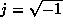,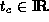is the center of the energy concentration in time,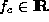is the center frequency,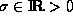is the spread of the pulse, and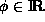is the phase shift of the wave, which we will not consider as one of the parameters. The subscripts of g represent the degrees of freedom, which comprise the parameter list.

We like the wave packet to have unit energy. Hence we reformulate the definition of the Gaussian envelope (taking advantage of the fact that a Gaussian function raised to any exponent, in our case, 1/2, is still a Gaussian function if multiplied by the appropriate normalization constant):

g^_t_c,f_c,(t) \!\!\! & \!\!\!=\!\!\! & \!\!\! 12(\!-12(t\!-\!t_c )^2 ) (j2f_c (t-t_c))
\!\!\! & \!\!\!=\!\!\! & \!\! 1_t (\!-12(t\!-\!t_c_t)^2 ) \!\! (j2f_c (t-t_c))

where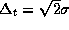.

Theoretically bandlimited signals have infinite duration, but it is customary, in electrical engineering, to use the 3dB bandwidth which is defined as the difference in frequencies, on either side of the peak, where the energy or power falls to half the peak value. This definition, however, is not theoretically motivated, nor particularly useful in our context. Therefore, in the case of the wave packet, we simply define the duration to be equal to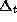in (2). By the reciprocal nature ofand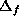, we are also implicitly specifying the bandwidth.

In (2), we can identify the Gaussian part as an envelope, which is modulated by a harmonic oscillation. The family of Gaussian chirplets is given by replacing the harmonic oscillation (wave) with a linear FM chirp:

g_t_c,f_c,(_t),c(t) = 1_t e^-12(t_t)^2 e^j2(c(t-t_c)^2 + f_c (t-t_c))

where we have used a logarithmic scale for the duration so that the unit width (default) is represented by a parameter of zero. Whenever a parameter is missing from the parameter list, we will assume it to be zero. For example, if only three parameters are present, we assume zero chirprate; if only two are present, we also assume that the log-duration is zero (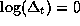). Summarizing, the Gaussian chirplet (3) has four parameters: time-center,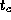; frequency-center,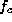; log-duration,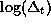; and chirprate, c.Next: Notation Up: THE CHIRPLET Previous: THE CHIRPLET

Steve Mann
Thu Jan 8 19:50:27 EST 1998